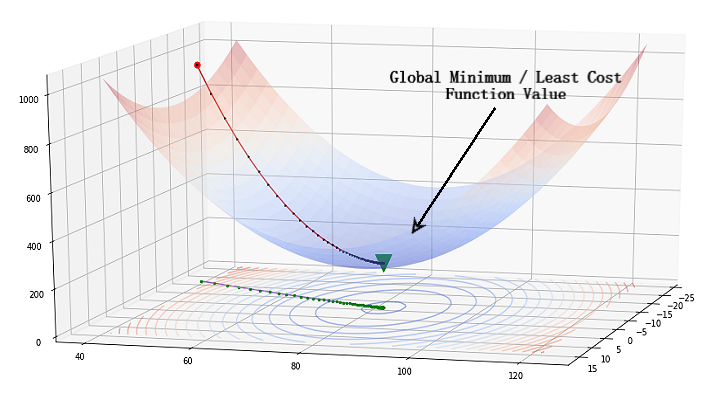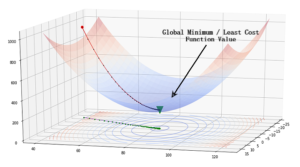# Simulated Annealing with C# Solving Optimization Problems with C

We will look at how to develop Simulated Annealing algorithm in C to find the best solution for an optimization problem.

The problem we are facing is that we need to construct a list from a given set of numbers (domain) provided that the list doesn’t have any duplicates and the sum of the list is equal to 13.

The full code can be found in the GitHub repo: https://github.com/MNoorFawi/simulated-annealing-in-c

## Defining the Problem

We have a domain which is the following list of numbers:

```// include the needed libraries
#include <stdio.h>
#include <stdlib.h>
#include <string.h>
#include <math.h>

// defining the available domain from which we get the answer
int domain = {1, 2, 3, 4, 5, 6, 7, 8, 9, 10, 11, 12, 13, 14, 15, 16, 17, 18, 19, 20};```

Our target is to construct a list of 4 members with no duplicates, i.e. unique numbers, and the sum of the list should be 13

Let’s define a couple of macros for these conditions

```#define CHOICE 20 // length of the domain
#define TARGET 13 // the target
#define LEN 4 // the length of the desired array```

Now we define some helper functions that will help in our program

```// returns random integer from 0 to n - 1
int rand_int(int n) {
int r, rand_max = RAND_MAX - (RAND_MAX % n);
while ((r = rand()) >= rand_max);
return r / (rand_max / n);
}

// print array
void print_arr(int * a, int l) {
int i = 0;
for (; i < l; ++i)
printf("%i ", a[i]);
}```

Cool!

Now as we have defined the conditions, let’s get into the most critical part of the algorithm. The cost function!

## Cost Function

The Cost Function is the most important part in any optimization algorithm. The algorithm searches different solutions in order to minimize the cost function of the current solution until it reaches the stop criteria.  The cost function is problem-oriented, which means we should define it according to the problem at hand, that’s why it is so important.

Our cost function for this problem is kind of simple. We can actually divide into two smaller functions; one to calculate the sum of the suggested list while the other checks for duplication.

```// the cost func here is to see if the array meets the target
int cost(int * a) {
int i, sum = 0;
for (i = 0; i < LEN; i++)
sum += a[i];
return sum;
}

// to ensure that all the values in the array are unique
int is_dup(int * a, int len) {
int i, j;
for (i = 0; i < len - 1; ++i) {
for (j = i + 1; j < len; ++j) {
if (a[i] == a[j])
return 1; // duplicates is found
}
}
return 0;
}```

We have now everything ready for the algorithm to start looking for the best solution.

## Simulated Annealing

Simulated annealing algorithm is an optimization method which is inspired by the slow cooling of metals. The algorithm starts with a random solution to the problem. It has a variable called temperature, which starts very high and gradually gets lower (cool down).

In each iteration, the algorithm chooses a random number from the current solution and changes it in a given direction. The cost is calculated before and after the change, and the two costs are compared. If the new cost is lower, the new solution becomes the current solution, just like any other optimization algorithm. However, if the cost is higher, the algorithm can still accept the current solution with a certain probability. This is to avoid the local minimum.

As the picture shows, the simulated annealing algorithm, like optimization algorithms, searches for the global minimum which has the least value of the cost function that we are trying to minimize.I prefer simulated annealing over gradient descent, as it can avoid the local minima while gradient descent can get stuck in it.

Now let’s define the algorithm:

```// simulated annealing algorithm
void siman(int * current_sol, int( * cost_func)(int * ), float temp, float cool) {
int i, j;
double current_cost, new_cost, p;
int new_sol[LEN];

// populate the initial solution randomly from the domain
for (i = 0; i < LEN; ++i)
current_sol[i] = domain[rand_int(CHOICE)];

// loop until temperature is cooled down totally
while (temp > 0.01) {
// choose a random element to update each iteration
i = rand_int(LEN);
// copy current_sol into new_sol
memcpy(new_sol, current_sol, sizeof(int) * LEN);
// update an element randomly from domain
new_sol[i] = domain[rand_int(CHOICE)];
// if array has duplicates skip
if (is_dup(new_sol, LEN))
continue;

// calculate cost of current solution and cost of new solution to compare
current_cost = cost_func(current_sol);
new_cost = cost_func(new_sol);
// a probability to help not looping forever
p = exp((-new_cost - current_cost) / temp);

// check if new cost has narrower gap with TARGET than the current cost
if (abs(TARGET - new_cost) < abs(TARGET - current_cost) || rand() < p) {
// if so then update current_sol to be the new solution
memcpy(current_sol, new_sol, LEN * sizeof(int));
printf("\ncurrent cost is: %f\n", new_cost);
puts("current solution is:");
print_arr(current_sol, LEN);
puts("");
}
// cool down
temp = temp * cool;
}
}
```

Perfect! We developed everything for the problem. Now let’s develop the program to test the algorithm

## C Main Function

We can easily now define a simple main() function and compile the code. But as you see, the siman function has arguments, temp and cool, that can usually be the same every run. So it would be better if we can make these arguments have default values.

C doesn’t support neither named nor default arguments. But with a little workaround, we can overcome this limitation and make our algorithm accept named arguments with default values.

We first define a struct which contains all the arguments:

```typedef struct {
int* current_sol;
int( * cost_func)(int * );
float temp, cool;
}
func_args;
```

Then, we define a wrapper function that checks for certain arguments, the default ones, if they are provided or not to assign the default values to them:

```void siman_wrapper(func_args input) {
/* our wrapper checks only for temp and cool
only and assign default values */
float temp = input.temp ? input.temp : 100.0;
float cool = input.cool ? input.cool : 0.99;
printf("\n Temperature = %.2f & Cool = %.2f\n", temp, cool);
return siman(input.current_sol, input.cost_func, temp, cool);
}```

Now we define a macro that the program will use, let’s say the macro will be the interface for the algorithm. The macro will convert input into the struct type and pass it to the wrapper which in turn checks the default arguments and then pass it to our siman algorithm.

```// macro for named args in a func
#define simulated_annealing(...) siman_wrapper((func_args) {__VA_ARGS__})```

Now comes the definition of our main program:

```int main() {
printf("\n\tTarget is an array of length %i unique values\n\tsum should be %i\n\tfrom numbers between 1 and %i\n",
LEN, TARGET, CHOICE);
// the array to be filled with possible answers
int * current_sol = (int * ) malloc(LEN * sizeof(int));
simulated_annealing(current_sol, cost);
return 0;
}```

## Compile and Run

At this point, we have done with developing, it is time to test that everything works well.

First we compile our program: I assume that you added all code in one file as in the github repo. However, you should feel free to have the project more structured into a header and .c files.

`gcc siman.c -o siman -lm`

‘-lm’ to include ‘math’ library

Then, we run the program and see the results:

```./siman

Target is an array of length 4 unique values
sum should be 13
from numbers between 1 and 20

Temperature = 100.00 & Cool = 0.99

current cost is: 45.000000
current solution is:
17 8 16 4

current cost is: 44.000000
current solution is:
17 8 15 4

...
...

current cost is: 12.000000
current solution is:
1 2 6 3

current cost is: 13.000000
current solution is:
1 2 6 4```

Great! It works so well.

You can also check how to develop simulated annealing algorithm in python to solve resource allocation